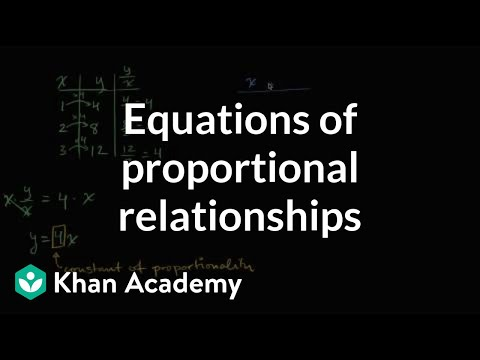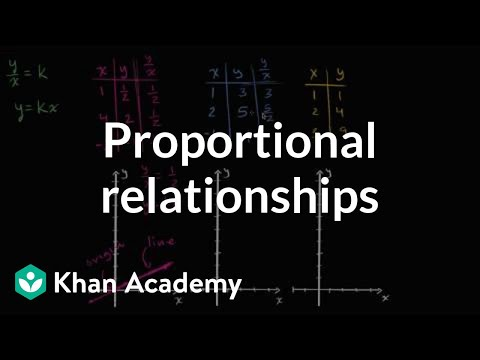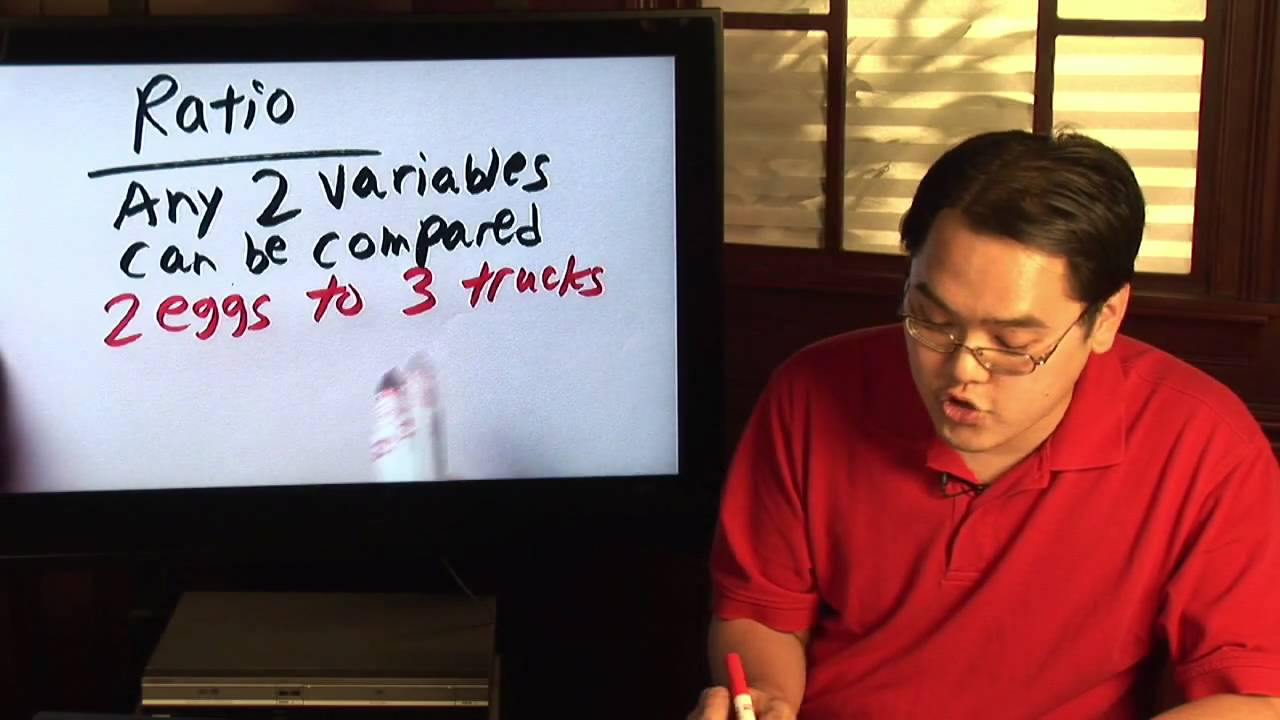# Writing proportional equations

##By Khan Academy

Learn how to write a proportional equation y=kx where k is the so-called constant of proportionality.# Identifying proportional relationships

##By Khan Academy

Is the volume of a square proportional to the side length of the square?# Identifying proportional relationships

##By Khan Academy

A proportionality problem about eating bananas# Identifying proportional relationships

##By Khan Academy

Given a table of ratios, watch as we test them for equivalence and determine whether the relationship is proportional.# Identifying proportional relationships

##By Khan Academy

The relationship between two variables is proportional ifï¿½ï¿½ï¿½ï¿½ï¿½ï¿½ï¿½ï¿½ï¿½ï¿½ï¿½ï¿½# Identifying proportional relationships

##By Khan Academy

Is the perimeter of a square proportional to the side length of the square?# Identifying proportional relationships with graphs

##By Khan Academy

Learn how to tell proportional relationships by drawing graphs. Key idea: the graph of a proportional relationship is a straight line through the origin.# Represent proportions by equations

##By Khan Academy

Proportional relationships# Significance of points on graphs of proportions

##By Khan Academy

Graphical representation# Interpreting graphs of proportional relationships

##By Khan Academy

Learn how to read and understand graphs of proportional relationships# Fractions & Proportions : Understanding Math Ratios

##By

In math, ratio is a fraction in which two variables can compared. Discover how to convert ratios into fractions with help from a math teacher in this free vi...# Triangles

##By MathPlanetVideos

Find the value of x in the triangle# Solving an Equation Involving Rational Exponents - Example 3

##By PatrickJMT

YouTube presents Solving an Equation Involving Rational Exponents - Example 3, an educational video resource on math.# Writing proportional equations

##By Khan Academy

Learn how to write a proportional equation y=kx where k is the so-called constant of proportionality.# Writing proportional equations

##By Khan Academy

Learn how to write a proportional equation y=kx where k is the so-called constant of proportionality.# Writing proportional equations

##By Khan Academy

Learn how to write a proportional equation y=kx where k is the so-called constant of proportionality.# Writing proportional equations

##By Khan Academy

Learn how to write a proportional equation y=kx where k is the so-called constant of proportionality.# Testing if Ratios are Proportional

##By Khan Academy

Watch this video to learn a method to test if two (or more) ratios are equal. Equal ratios are called proportional. If the ratios can be reduced the same fraction in lowest terms then they are proportional.# Fractions & Proportions : Understanding Math Ratios

##By eHow

YouTube presents Fractions & Proportions : Understanding Math Ratios an educational video resources on math.# Interpreting graphs of proportional relationships

##By Khan Academy

Learn how to read and understand graphs of proportional relationships